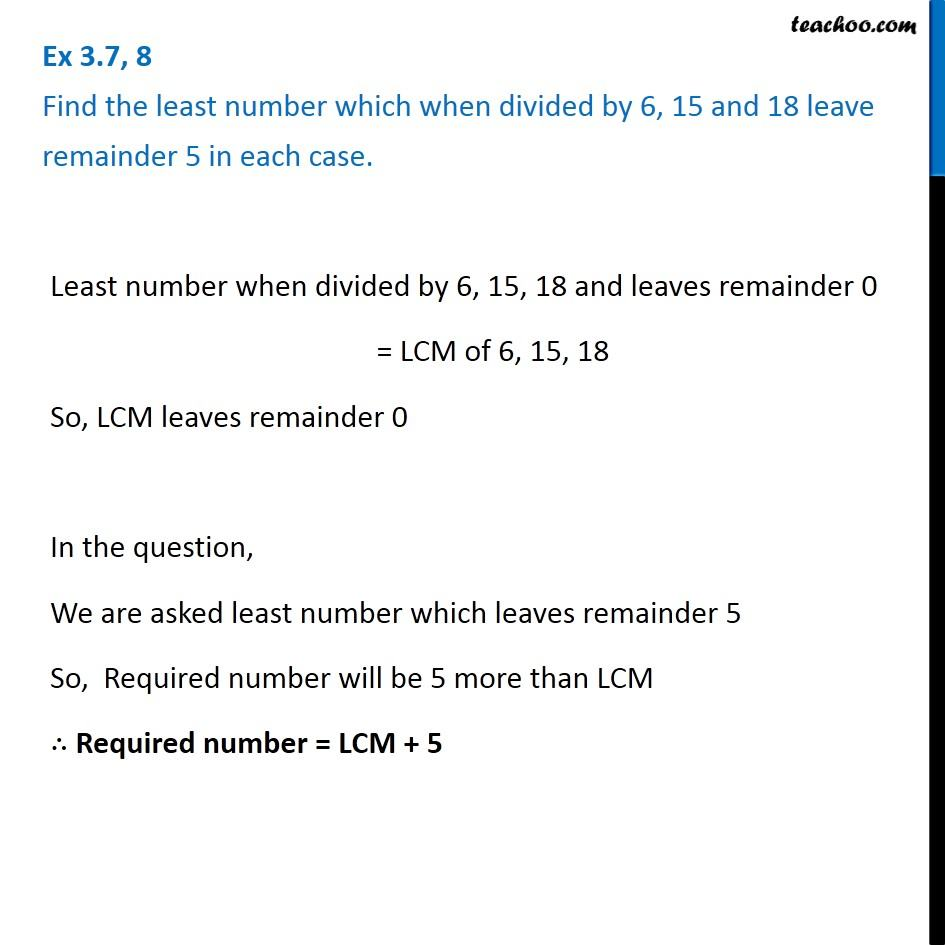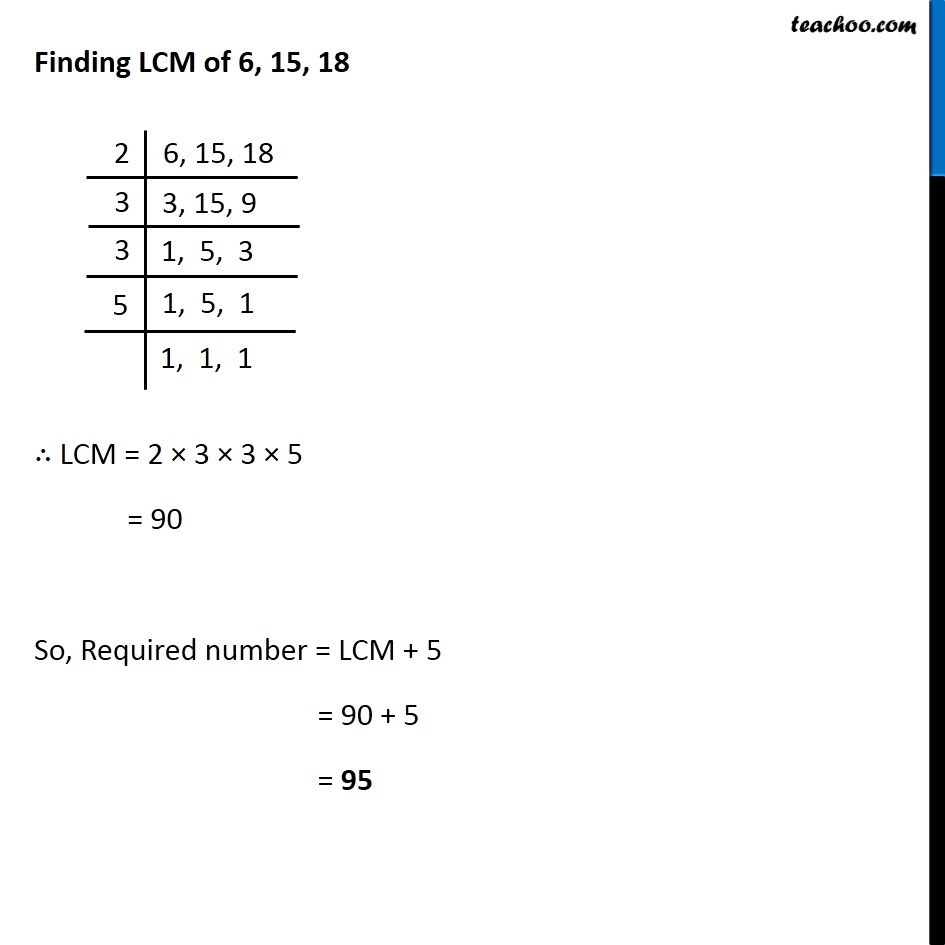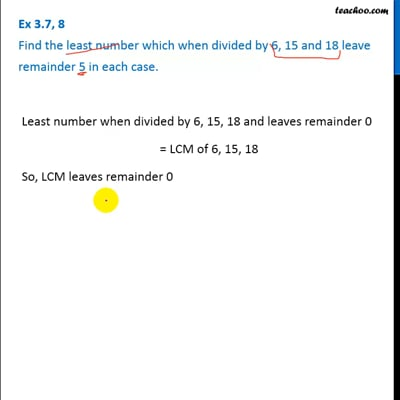Ex 3.7

Chapter 3 Class 6 Playing with Numbers
Serial order wiseThis video is only available for Teachoo black users

Learn in your speed, with individual attention - Teachoo Maths 1-on-1 Class

### Transcript

Least number when divided by 6, 15, 18 and leaves remainder 0 = LCM of 6, 15, 18 So, LCM leaves remainder 0 In the question, We are asked least number which leaves remainder 5 So, Required number will be 5 more than LCM ∴ Required number = LCM + 5 ∴ LCM = 2 × 3 × 3 × 5 = 90 So, Required number = LCM + 5 = 90 + 5 = 95